# Filter Design for Up- and Downconversion¶

In the previous notebook, we have simply chosen some filter for the DA and AD conversion, without further evaluation. For most situations, the chosen filter is fine, though let us still look a bit more detailed at this filter and its effects. In particular we will look at the signal spectrum after upconversion and DA conversion, where we will see interesting effects. The content of this notebook is especially important for the notebook 09 - OFDM-Related and 5G Waveforms.ipynb and you can safely omit it on your first exploration.

First, let's import common objects:

In :
from audioComms.components import Component, TX, Environment, Recorder
from audioComms.utils import get_filter, StatefulFilter
from audioComms.plotting import PlotWaveform, PlotSpectrum
from audioComms.channels import SimulatedChannel, AudioChannel
from audioComms.passband import InterpolationUpconversion, DownconversionDecimation, PassbandChannel


Note the PassbandChannel class, which combines Upconversion, actual channel and Downconversion into a single object. We will use this channel often to simplify the simulation code.

Below, we write a function that shows the time domain and spectrum of the upconverted transmit signal of any given signal generator:

In :
def showTXSpectrum(txFunc, AD_DA_filterLength=3, AD_DA_filterUseWindow=False):
Fc = 10000
B = 441*5*4
Fs = 44100
env = Environment(samplerate=Fs, simulationDuration=10)

tx = txFunc(env)

fig = env.figure(figsize=(8,3))
showSignal = PlotWaveform(env, duration=1, numLines=1, axes=fig.add_subplot(121), ylim=(-2,2))
showSpectrum = PlotSpectrum(env, windowDuration=0.5, axes=ax, logScale=True, xlim=(0, Fs/2), ylim=(-100, -30))
ax.axvline(Fc-B/2, ls='--', color='r'); ax.axvline(Fc+B/2, ls='--', color='r')

tx.transmitsTo(chan)
chan.transmitsTo(showSignal, stream='TxRF')
chan.transmitsTo(showSpectrum, stream='TxRF')

env.run()


Let's try this function out using a white noise transmitter. This transmitter just generates complex white noise. Accordingly, the its spectrum should be flat over the whole bandwidth.

In :
class NoiseTransmitter(TX):
def __init__(self, environment):
super().__init__(environment)

def _generateSignal(self):
return (np.random.randn(1000)+1j*np.random.randn(1000))


First, let's verify this by concatenating some signal blocks and looking at its baseband spectrum:

In :
NT = NoiseTransmitter(None)
signal = np.hstack([NT._generateSignal() for _ in range(1000)])
H = np.fft.fft(signal) / np.sqrt(len(signal))
f = np.linspace(-0.5, 0.5, len(H), endpoint=False)
plt.figure(figsize=(8,3))
plt.plot(f, 20*np.log10(np.fft.fftshift(abs(H)))); plt.xlim((-0.5,0.5))
plt.xlabel('Normalized frequency'); plt.ylabel('$|H(f)|$ [dB]');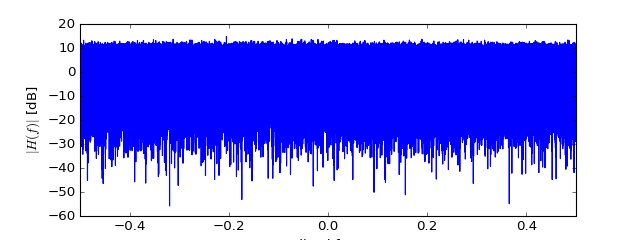Yes, in the baseband the transmit spectrum is completely flat. Let's look at the transmit spectrum after DA conversion and upconversion:

In :
showTXSpectrum(NoiseTransmitter)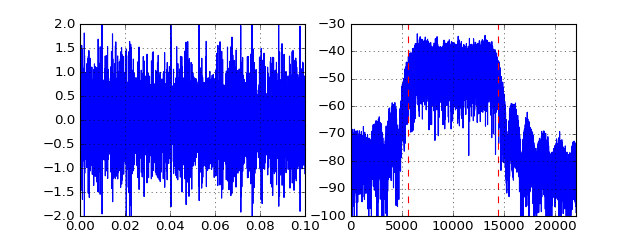Stop received from external


Hm... there is not really a flat spectrum. OK, within the red lines (i.e. the baseband bandwidth) the spectrum is almost flat as we saw before. However, there are significant sidelobes in other areas of the spectrum. Where do they come from? Let us recap the implementation of the AD and DA filter:

In :
# Source code has been redacted in online version
# Omitting 9 lines of source code


In above implementation, we have already added some parameters to tune the filter parameters. Let us write a function to look at the temporal and spectral properties of the DA/AD filter:

In :
def showDA_FilterDesign(filterLength=3, useWindow=False):
Fs = 44100
B = 441*5*4
Fc = 10000
F = UpDownConversionFilter(B=B, samplerate=Fs, N=filterLength, useWindow=useWindow)
plt.figure(figsize=(8,4))
plt.subplot(211)
t = (np.arange(len(F._b))-len(F._b)/2) / Fs * B
plt.plot(t, F._b); plt.grid(True)

plt.subplot(212)
H = np.fft.fft(F._b, len(F._b)*19) / Fs*B # perform oversampled fft
f = Fc+np.linspace(-Fs/2, Fs/2, len(H), endpoint=False)
plt.plot(f, 20*np.log10(np.fft.fftshift(abs(H)))); plt.grid(True)
plt.axvline(Fc+B/2, ls='--', color='r'); plt.axvline(Fc-B/2, ls='--', color='r');
plt.ylim((-100, 0)); plt.xlim((0, Fs/2));

In :
showDA_FilterDesign()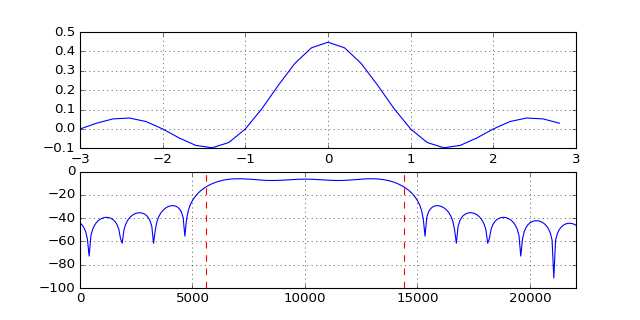Uh, the filter we have designed also shows the sidelobes at exactly the same positions as in the RX spectrum. Looking at the impulse response of the filter, these side lobes become clear: The filter is just abruptly stopping in the time domain, making it virtually windowed by a rectangular window. Let us try to see what happens when we use a different window (in this case it's a Kaiser window):

In :
showDA_FilterDesign(useWindow=True)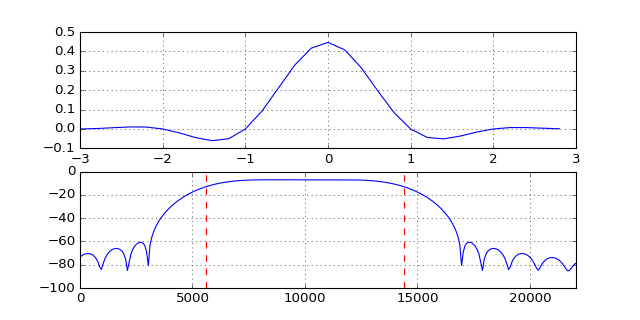Great! The sidelobes significantly decrease. However, as the downside the main lobe becomes much wider, reaching significantly outside of the baseband bandwidth. Let's see, if this behaviour is also occuring in the transmitted signal:

In :
showTXSpectrum(NoiseTransmitter, AD_DA_filterUseWindow=True)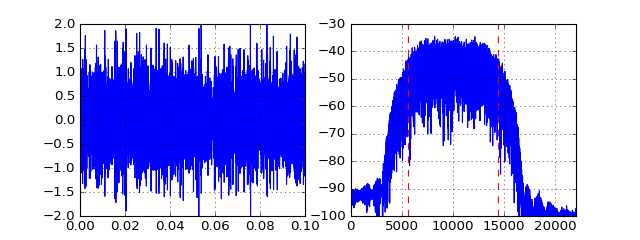Stop received from external


Yes, the sidelobes have decayed significantly, but the overall signal became much wider than the actual baseband bandwidth. Maybe, this is also not what we want. What can we do to reduce both the sidelobes and keep the signal spectrally confined? We can approximate the underlying infinitely long RRC filter more accurately by using a longer interpolation filter. For example, let's use a filter that has the length of 9 baseband samples and again look at its spectrum:

In :
showDA_FilterDesign(filterLength=9, useWindow=True)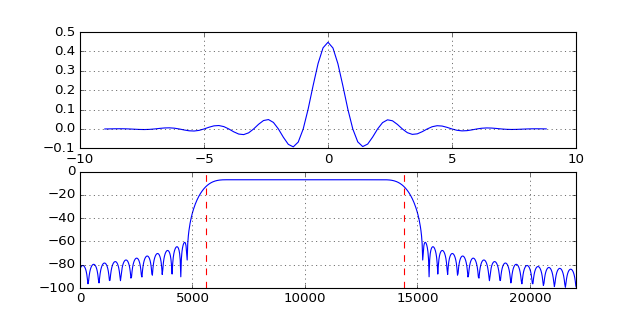Great! This filter has low sidelobes but also does not widen the mainlobe too much. At the downside, the longer filter requires more calculations to perform the interpolation operation and hence costs more operation power. For completeness, let's use this filter in the RF signal transmission:

In :
showTXSpectrum(NoiseTransmitter, AD_DA_filterLength=9, AD_DA_filterUseWindow=True)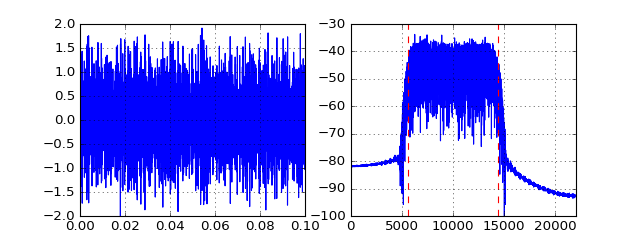Stop received from external


Yes, again the RF spectrum follows our analysis of the DA filter. Using this filter we have a narrow TX spectrum where at the same time only very low sidelobes occur.

# Conclusion¶

In this notebook we have touched the filter design for our up- and downconversion units. We have seen that using a window and a longer filter response improves the spectral properties, however at the cost of increased calculation complexity. We will need the presented knowledge in the notebook about 5G Waveforms. The next notebook is about fundamentals of OFDM modulation and demodulation.